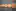# Order Notion chart by date

Tags
Tips
Formula
Template
Published
Oct 22, 2021
How to order my notion chart by date ?
That's a question that we often get at Nochart. So we though that this would be an interesting article for you guys :)

## Ordering your chart by date

Most of the time, ordering a notion chart by date in Nochart is straightforward to do:
• Select the date you want to order by :

## Ordering your chart when your column is not a date

But sometime, your month property in Notion is not set as a date.
When your date is set as a select or a text, Nochart won't detect that it's actually a date and will treat it as a text and sort it alphabetically.
``if(prop("Month text") == "January", 1, if(prop("Month text") == "February", 2, if(prop("Month text") == "March", 3, if(prop("Month text") == "April", 4, if(prop("Month text") == "May", 5, if(prop("Month text") == "June", 6, if(prop("Month text") == "July", 7, if(prop("Month text") == "August", 8, if(prop("Month text") == "September", 9, if(prop("Month text") == "October", 10, if(prop("Month text") == "Novermber", 11, if(prop("Month text") == "December", 12, 1))))))))))))``
``dateAdd(dateAdd(dateAdd(fromTimestamp(0), 1 - 1, "days"), prop("Calculated Month") - 1, "months"), ((prop("Calculated Month") > month(now())) ? year(now()) : toNumber(prop("Year"))) - 1970, "years")``